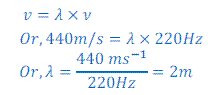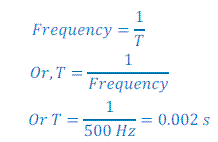# Sound, NCERT Solutions, Class 9, Science Notes - Class 9

## Class 9: Sound, NCERT Solutions, Class 9, Science Notes - Class 9

The document Sound, NCERT Solutions, Class 9, Science Notes - Class 9 is a part of Class 9 category.
All you need of Class 9 at this link: Class 9

## NCERT Book Class Nine In Text Questions and Answers

Page 162

Question: 1 - How does the sound produced by a vibrating object in a medium reach your ear?

Answer:- Vibrations in an object create disturbance in the medium and consequently compressions and rarefactions. Because of these compressions and rarefactions sound reaches to our ear.

Page 163

Question: 1 - Explain how sound is produced by your school bell.

Answer:- School bell starts vibrating when heated which creates compression and rarefaction in air and sound is produced.

Question: 2 - Why are sound waves called mechanical waves?

Answer: Since sound waves do some mechanical work while making disturbance in medium, hence sound waves are called mechanical wave.

Question: 3 -Suppose you and your friend are on the moon. Will you be able to hear any sound produced by your friend?

Answer: Since sound waves require medium to propagate and there is no medium present on the moon. So, I will not able to hear the sound of my friend on the moon.

Page 165

Question: 1 - Question: 1 - Which wave property determines (a) loudness, (b) pitch?

Answer: (a) Amplitude of sound waves determines loudness. Louder sound has greater amplitude and vice versa.

(b) Frequency of the sound waves determined pitch of the sound.

Question: 2 - Guess which sound has a higher pitch: guitar or car horn?

Answer: Sound of the car horn has higher pitch.

Question: 3 - What are wavelength, frequency, time period and amplitude of a sound wave?

Answer: Wavelength: Wavelength is the distance between two consecutive compressions or rarefaction of wave.

Frequency: The number of sound wave produced in one second is called frequency.

Time period: Time period is the time taken to produce one wave of sound.

Amplitude: Amplitude is the maximum displacement along the mean position of the particles of medium.

Question: 4 - How are the wavelength and frequency of a sound wave related to its speed?

Answer: The relation between frequency and wavelength of sound wave is given as follows:

Velocity (v)=Wavelength(λ)X Frequency (ν), v = λX ν

This means the speed is equal to the product of wavelength and frequency of the sound wave.

This equation is also called the ‘wave equation’ and applicable to all types of wave.

Question: 5 - Calculate the wavelength of a sound wave whose frequency is 220 Hz and speed is 440 m/s in a given medium.

Given,

Frequency (ν) = 220 Hz

Velocity (v) = 440m/s

Wavelength (λ) =?

We know;Thus, wavelenght = 2m

Question: 6 - A person is listening to a tone of 500 Hz sitting at a distance of 450 m from the source of the sound. What is the time interval between successive compressions from the source?

Answer: Since, the time interval between successive compressions is called time period or time interval.

Here given,

Frequency (ν) = 500Hz

T (Time period) =?

We know that;Thus, time interval between two consecutive compression of the given wave = 0.2 s

Question: 7 - Distinguish between loudness and intensity of sound.

Answer: Loudness of sound is determined of amplitude and intensity of the sound wave is determined by frequency of sound waves.

Page 167

Question: 1 - In which of the three media; air, water or iron does the sound travel the fastest at a particular temperature?

Answer:At particular temperature sound travels fastest in iron.

Page 168

Question: 1 - An echo returned in 3 s. What is the distance of the reflecting surface from the source, given that the speed of sound is 342 m /s?

Answer:To return an echo sound has to cover distance of two way.

Here, given,

Speed of sound = 342 m/s

Time = 3s

Thus,

Distance = speed X time

⇒ Distance = 342m/s×3 s=1026 m

Thus,the distance between the source and reflecting surface=1026÷2=513m

Page 169

Question: 1 -Why are the ceilings of concert halls curved?

Answer: Since, concert halls are big, so audience at the back rows of the hall may not hear clear sound of speaker. To overcome this problem, the ceiling of the concert halls is made concave. Concave ceiling helps the sound wave to reflect and send to farther distance which makes the concert hall enable to send clear sound to the audience even sitting in back rows of hall.

Page 170

Question: 1 - What is the audible range of the average human ear?

Answer: 20 Hz to 20000 Hz

Question: 2 - What is the range of frequencies associated with

(a) Infrasound

(b) Ultrasound

(a) Infrasound: Less than 20 Hz

(b) Ultrasound: More than 20000 Hz

Page 172

Question: 1 - A submarine emits a SONAR pulse, which returns from an underwater cliff in 1.02 s. If the speed of sound in salt water is 1531 m/s, how far away is the cliff ?

Answer:To return the SONAR pulse back, its wave has to travel two way.

Here, given,

Velocity (v) of sound wave = 1531m/s

Time (T) = 1.02 s

Thus, Distance = speed X time

Distance=1531 ms^(-1)×1.02 s=1561.62 m

So,the distance between the source and reflecting surface=1561.62÷2=780.81m

The document Sound, NCERT Solutions, Class 9, Science Notes - Class 9 is a part of Class 9 category.
All you need of Class 9 at this link: Class 9Use Code STAYHOME200 and get INR 200 additional OFF

Track your progress, build streaks, highlight & save important lessons and more!

,

,

,

,

,

,

,

,

,

,

,

,

,

,

,

,

,

,

,

,

,

,

,

,

,

,

,

,

,

,

;